# 1 Introduction

Single-cell RNA sequencing (scRNA-Seq) technologies are opening the way for transcriptome-wide profiling across diverse and complex mammalian tissues, facilitating unbiased identification of novel cell sub-populations and discovery of novel cellular function. As in other high-throughput analyses, a large fraction of the variability observed in scRNA-Seq data results from batch effects and other technical artifacts (Hicks, Teng, and Irizarry 2015). In particular, a unique reliance on minuscule amounts of starting mRNA can lead to widespread “drop-out effects,” in which expressed transcripts are missed during library preparation and sequencing. Due to the biases inherent to these assays, data normalization is an essential step prior to many downstream analyses. As we face a growing cohort of scRNA-Seq technologies, diverse biological contexts, and novel experimental designs, we cannot reasonably expect to find a one-size-fits-all solution to data normalization.

`scone` supports a rational, data-driven framework for assessing the efficacy of various normalization workflows, encouraging users to explore trade-offs inherent to their data prior to finalizing their data normalization strategy. We provide an interface for running multiple normalization workflows in parallel, and we offer tools for ranking workflows and visualizing study-specific trade-offs.

This package was originally developed to address normalization problems specific to scRNA-Seq expression data, but it should be emphasized that its use is not limited to scRNA-Seq data normalization. Analyses based on other high-dimensional data sets - including bulk RNA-Seq data sets - can utilize tools implemented in the `scone` package.

## 1.1 Human Neurogenesis

We will demonstrate the basic `scone` workflow by using an early scRNA-Seq data set (Pollen et al. 2014). We focus on a set of 65 human cells sampled from four biological conditions: Cultured neural progenitor cells (“NPC”) derived from pluripotent stem cells, primary cortical samples at gestation weeks 16 and 21 (“GW16” and “GW21” respectively) and late cortical samples cultured for 3 weeks (“GW21+3”). Gene-level expression data for these cells can be loaded directly from the `scRNAseq` package on Bioconductor.

``````library(scRNAseq)

## ----- Load Example Data -----
fluidigm <- ReprocessedFluidigmData(assays = "rsem_counts")``````

The `rsem_counts` assay contains expected gene-level read counts via RSEM (Li and Dewey 2011) quantification of 130 single-cell libraries aligned to the hg38 RefSeq transcriptome. The data object also contains library transcriptome alignment metrics obtained from Picard and other basic tools.

``````## ----- List all QC fields -----

# List all qc fields (accessible via colData())
``````##   "NREADS"                       "NALIGNED"
##   "RALIGN"                       "TOTAL_DUP"
##   "PRIMER"                       "INSERT_SZ"
##   "INSERT_SZ_STD"                "COMPLEXITY"
##   "NDUPR"                        "PCT_RIBOSOMAL_BASES"
##  "PCT_CODING_BASES"             "PCT_UTR_BASES"
##  "PCT_INTRONIC_BASES"           "PCT_INTERGENIC_BASES"
##  "PCT_MRNA_BASES"               "MEDIAN_CV_COVERAGE"
##  "MEDIAN_5PRIME_BIAS"           "MEDIAN_3PRIME_BIAS"
##  "MEDIAN_5PRIME_TO_3PRIME_BIAS"``````

All cell-level metadata, such as cell origin and sequence coverage (“low” vs “high” coverage) can be accessed using `colData()`:

``````# Joint distribution of "biological condition"" and "coverage type""
table(colData(fluidigm)\$Coverage_Type,
colData(fluidigm)\$Biological_Condition)``````
``````##
##        GW16 GW21 GW21+3 NPC
##   High   26    8     16  15
##   Low    26    8     16  15``````

Each cell had been sequenced twice, at different levels of coverage. In this vignette we will focus on the high-coverage data. Before we get started we will do some preliminary filtering to remove the low-coverage replicates and undetected gene features:

``````# Preliminary Sample Filtering: High-Coverage Only
is_select = colData(fluidigm)\$Coverage_Type == "High"
fluidigm = fluidigm[,is_select]

# Retain only detected transcripts
fluidigm = fluidigm[which(apply(assay(fluidigm) > 0,1,any)),]``````

## 1.2 Visualizing Technical Variability and Batch Effects

One of our alignment quality readouts is the fraction of reads aligned to the transcriptome. We can use simple bar plots to visualize how this metric relates to the biological batch.

``````# Define a color scheme
cc <- c(brewer.pal(9, "Set1"))

# One batch per Biological Condition
batch = factor(colData(fluidigm)\$Biological_Condition)

# Alignment Quality Metrics

# Barplot of read proportion mapping to human transcriptome
ralign = qc\$RALIGN
o = order(ralign)[order(batch[order(ralign)])] # Order by batch, then value

barplot(ralign[o], col=cc[batch][o],
legend("bottomleft", legend=levels(batch), fill=cc,cex=0.4)``````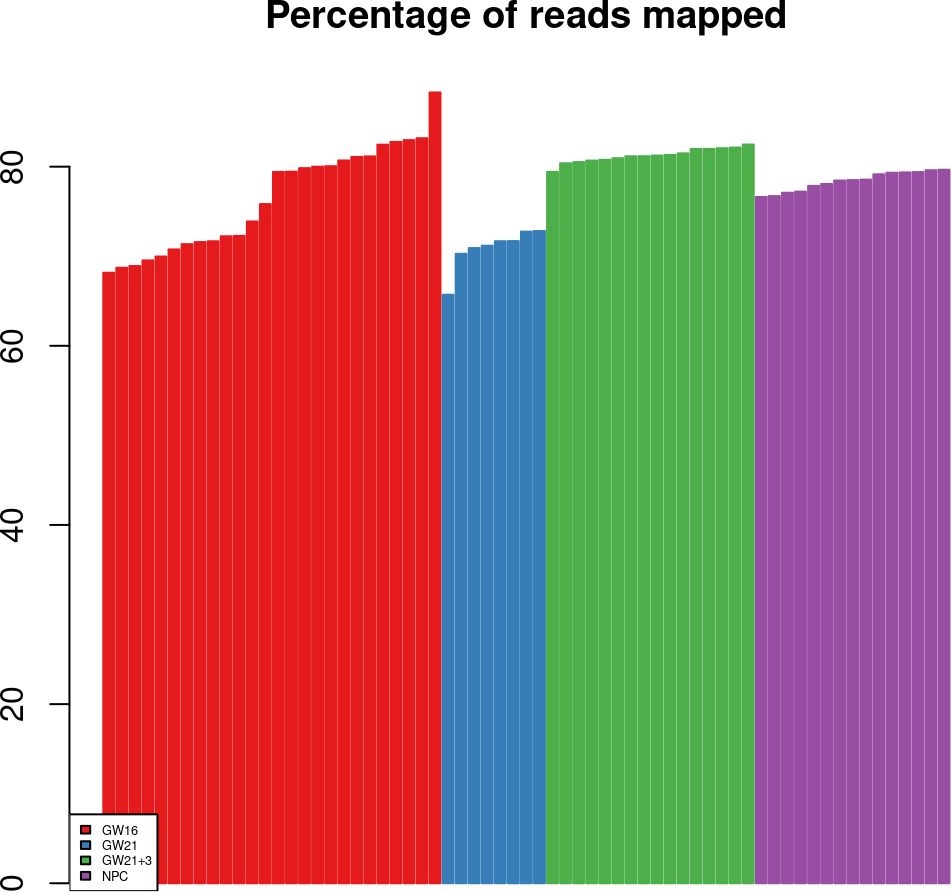We can see modest differences between batches, and we see that there is one GW21 cell with a particularly low alignment rate relative to the rest of the GW21 batch. These types of observations can inform us of “poor-quality” libraries or batches. We may alternatively consider the number of reads for each library:

``````# Barplot of total read number

legend("topright", legend=levels(batch), fill=cc, cex=0.4)``````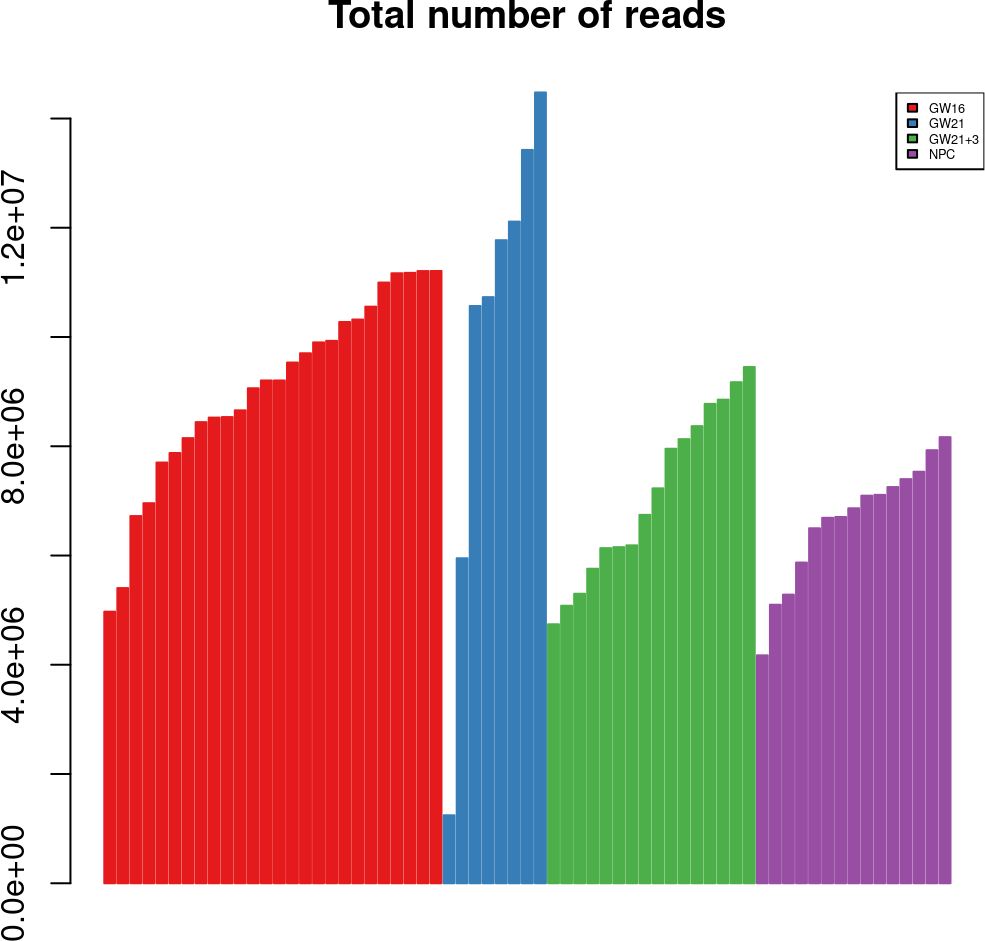We see that read coverage varies substantially between batches as well as within batches. These coverage differences and other technical features can induce non-intuitive biases upon expression estimates. Though some biases can be addressed with simple library-size normalization and cell-filtering, demand for greater cell numbers may require more sophisticated normalization methods in order to compare multiple batches of cells. Batch-specific biases are impossible to address directly in this study as biological origin and sample preparation are completely confounded.

While it can be very helpful to visualize distributions of single quality metrics it should be noted that QC metrics are often correlated. In some cases it may be more useful to consider Principal Components (PCs) of the quality matrix, identifying latent factors of protocol variation:

``````## ----- PCA of QC matrix -----
qpc = prcomp(qc,center = TRUE,scale. = TRUE)
barplot((qpc\$sdev^2)/sum(qpc\$sdev^2), border="gray",
xlab="PC", ylab="Proportion of Variance", main="Quality PCA")``````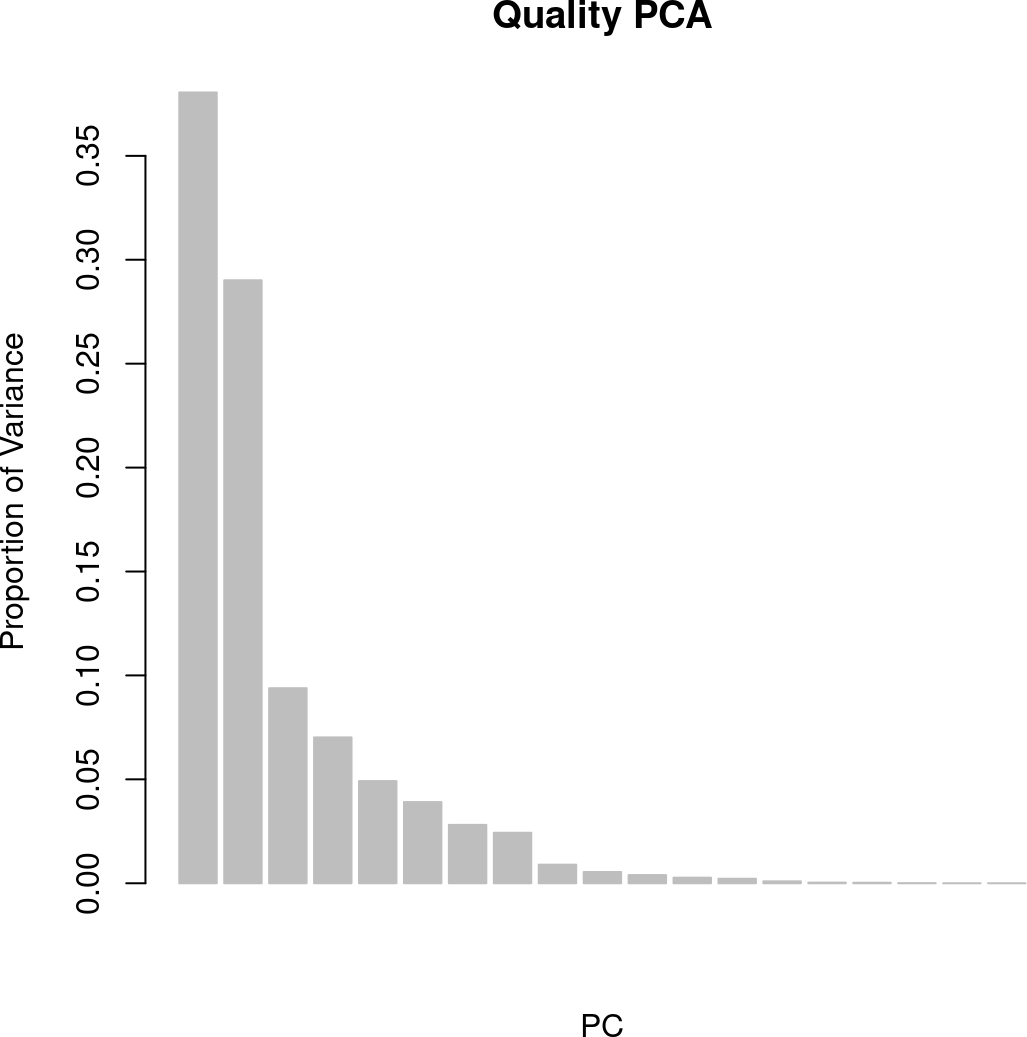Even though 19 different QC metrics have been quantified in this analysis, PCA shows us that only a small number of PCs are needed to described a majority of the QC variance (e.g. 3 to explain 76%). We will now visualize the distribution of the first PC in the context of batch:

``````# Barplot of PC1 of the QC matrix
qc1 = as.vector(qpc\$x[,1])
o = order(qc1)[order(batch[order(qc1)])]

barplot(qc1[o], col=cc[batch][o],
border=cc[batch][o], main="Quality PC1")
legend("bottomright", legend=levels(batch),
fill=cc, cex=0.8)``````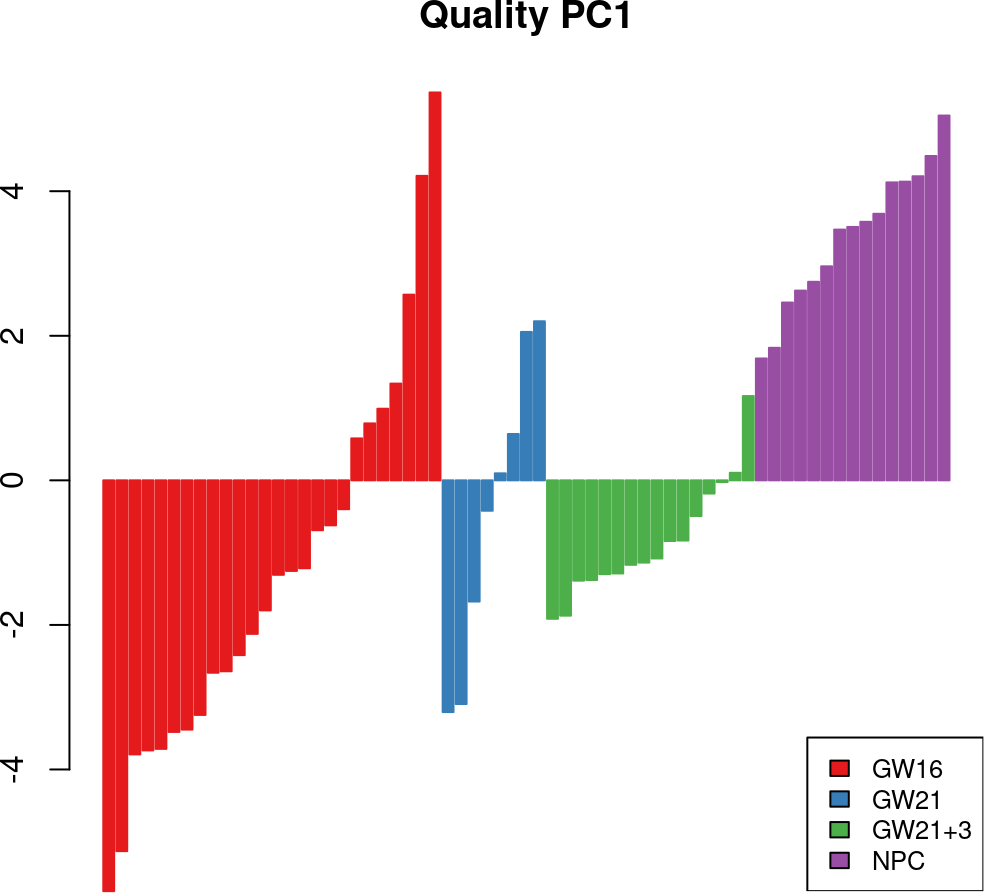This first PC appears to represent both inter-batch and intra-batch sample heterogeneity, similar the the total number of reads. If this latent factor reflects variation in sample preparation, we may expect expression artifacts to trace this factor as well: in other words, we should be very skeptical of genes for which expression correlates strongly with the first PC of quality metrics. In this vignette we will show how latent factors like this can be applied to the normalization problem.

## 1.3 Drop-out Characteristics

Before we move on to normalization, let’s briefly consider a uniquely single-cell problem: “drop-outs.” One of the greatest challenges in modeling drop-out effects is modeling both i) technical drop-outs and ii) biological expression heterogeneity. One way to simplify the problem is to focus on genes for which we have strong prior belief in true expression. The `scone` package contains lists of genes that are believed to be ubiquitously and even uniformly expressed across human tissues. If we assume these genes are truly expressed in all cells, we can label all zero abundance observations as drop-out events. We model detection failures as a logistic function of mean expression, in line with the standard logistic model for drop-outs employed by the field:

``````# Extract Housekeeping Genes
data(housekeeping)
hk = intersect(housekeeping\$V1,rownames(assay(fluidigm)))

# Mean log10(x+1) expression
mu_obs = rowMeans(log10(assay(fluidigm)[hk,]+1))

# Assumed False Negatives
drop_outs = assay(fluidigm)[hk,] == 0

# Logistic Regression Model of Failure
ref.glms = list()
for (si in 1:dim(drop_outs)){
fit = glm(cbind(drop_outs[,si],1 - drop_outs[,si]) ~ mu_obs,
family=binomial(logit))
ref.glms[[si]] = fit\$coefficients
}``````

The list `ref.glm` contains the intercept and slope of each fit. We can now visualize the fit curves and the corresponding Area Under the Curves (AUCs):

``````par(mfrow=c(1,2))

# Plot Failure Curves and Calculate AUC
plot(NULL, main = "False Negative Rate Curves",
ylim = c(0,1),xlim = c(0,6),
ylab = "Failure Probability", xlab = "Mean log10 Expression")
x = (0:60)/10
AUC = NULL
for(si in 1:ncol(assay(fluidigm))){
y = 1/(exp(-ref.glms[[si]] - ref.glms[[si]] * x) + 1)
AUC[si] = sum(y)/10
lines(x, 1/(exp(-ref.glms[[si]] - ref.glms[[si]] * x) + 1),
type = 'l', lwd = 2, col = cc[batch][si])
}

# Barplot of FNR AUC
o = order(AUC)[order(batch[order(AUC)])]

barplot(AUC[o], col=cc[batch][o], border=cc[batch][o], main="FNR AUC")
legend("topright", legend=levels(batch), fill=cc, cex=0.4)``````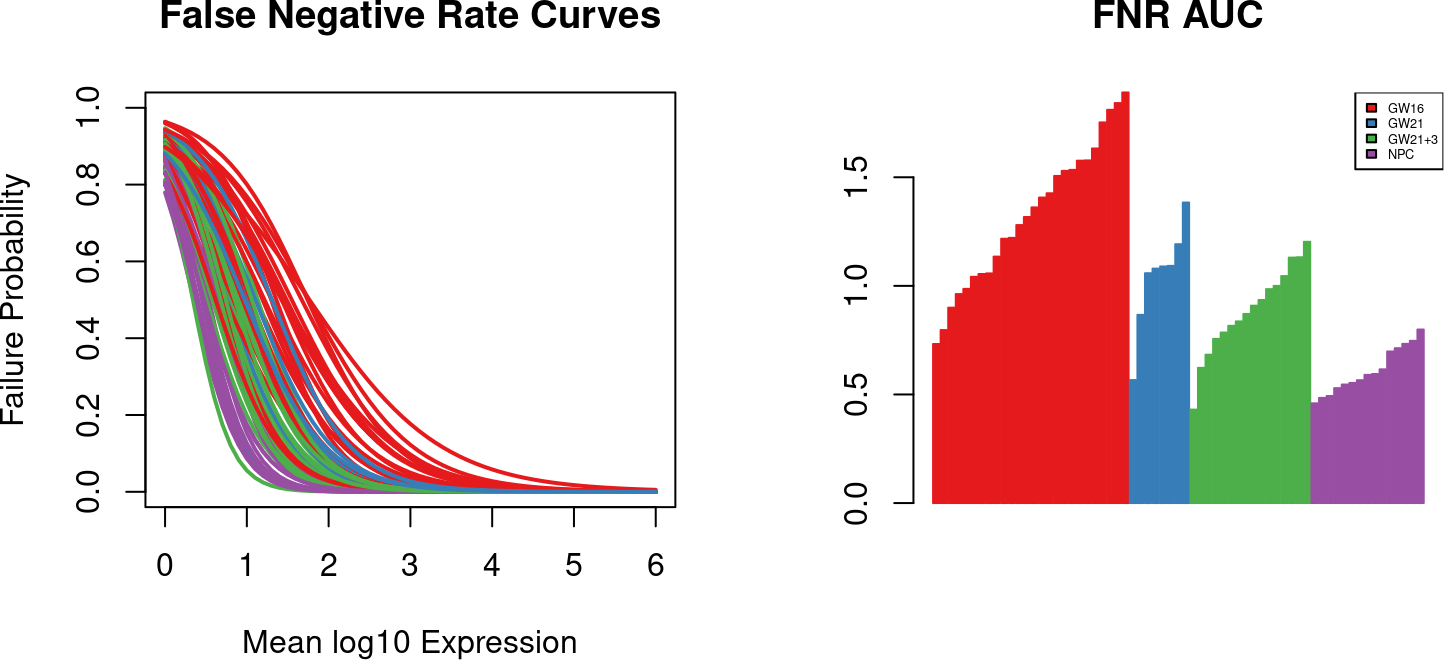Model-based metrics such as these may be more interpretable with respect to upstream sample preparation, and can be very useful for assessing single-cell library quality.

## 1.4 The `scone` Workflow

So far we have only described potential problems with single-cell expression data. Now we will take steps to address problems with our example data set. The basic QC and normalization pipeline we will use in this vignette allows us to:

• Filter out poor libraries using the `metric_sample_filter` function.
• Run and score many different normalization workflows (different combinations of normalization modules) using the main `scone` function.
• Browse top-ranked methods and visualize trade-offs with the `biplot_color` and `sconeReport` function.

In order to run many different workflows, SCONE relies on a normalization workflow template composed of 3 modules:

1. Data imputation: replacing zero-abundance values with expected values under a prior drop-out model. As we will see below, this module may be used as a modifier for module 2, without passing imputed values forward to downstream analyses. 2) Scaling or quantile normalization: either i) normalization that scales each sample’s transcriptome abundances by a single factor or ii) more complex offsets that match quantiles across samples. Examples: TMM or DESeq scaling factors, upper quartile normalization, or full-quantile normalization.
2. Regression-based approaches for removing unwanted correlated variation from the data, including batch effects. Examples: RUVg (Risso et al. 2014) or regression on Quality Principal Components described above.

# 2 Sample Filtering with `metric_sample_filter`

The most basic sample filtering function in `scone` is the `metric_sample_filter`. The function takes a consensus approach, retaining samples that pass multiple data-driven criteria.

`metric_sample_filter` takes as input an expression matrix. The output depends on arguments provided, but generally consists of a list of 4 logicals designating each sample as having failed (TRUE) or passed (FALSE) threshold-based filters on 4 sample metrics:

• Ratio of reads aligned to the genome. Requires the `ralign` argument.
• “Transcriptome breadth” - Defined here as the proportion of “high-quality” genes detected in the sample. Requires the `gene_filter` argument.
• FNR AUC. Requires the `pos_controls` argument.

If required arguments are missing for any of the 4, the function will simply return NA instead of the corresponding logical.

``````# Initial Gene Filtering:
# Select "common" transcripts based on proportional criteria.